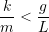### Review of single and multi-degree of freedom (mdof) systems: Introduction and Review

We are interested in small motions about some equilibrium positions. For SDOF systems,is usually measured from this static equilibrium position.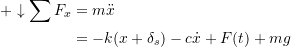Let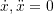for static equilibrium. Therefore: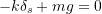Let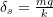. Therefore: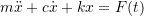All SDOF systems can be reduced to this form (assuming viscous damping):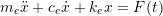Wheremeans equivalent. To determine the nature of the motion, first consider this linearized equation of free vibration (Note: May have to linearize).

Assume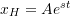(homogeneous), where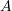is constant andis to be determined.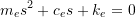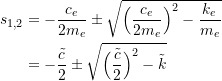If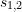are real and negative, then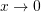as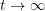. If either of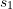or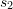are real and positive, then the solution increases with time.

Ifare complex, then they are complex conjugates and the nature of the motion depends on the real part. If the real part is negative,as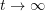. If positive,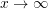as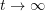. If the real part is zero, the motion is oscillatory and this is the borderline case between stability and instability.

Consider all cases:

1.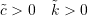. The roots have a negative real part and is stable regardless of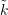. If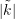is larger than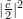, there will be an oscillatory part, but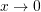as. This is called asymptotically stable.
2.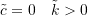. The roots are imaginary, and the system is borderline stable.
3.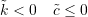. The roots have a positive real part, or are complex conjugates. Therefore, the system is unstable.
4.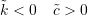One root has a positive real part. Therefore the system is unstable. The damping cannot overcome the negative spring.
5.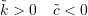. The roots have a positive real part, and therefore the system is unstable. Negative damping feeds energy into the system.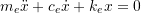Drop, but remember that,,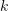are equivalent. Thus: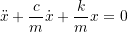Again, assume that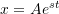. Thus: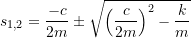The critical value is then when: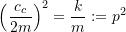Alternatively: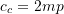Define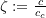. Then: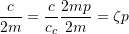Thus: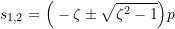Where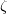is the damping ratio (system parameter).

Assuming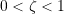: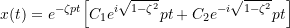And using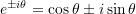: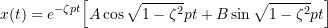Using initial conditions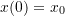,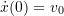, we get the following: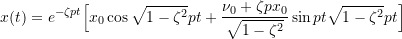We can write this as: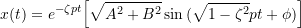Where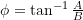,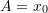, and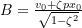.

In some problems, we have to find the equilibrium (static) configurations and then determine the motion about these positions.

#### Example

Find the motion about the equilibrium configuration.slides on a wire with viscous friction.

##### Solution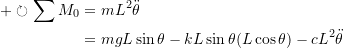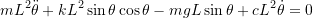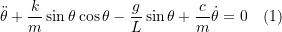We must find static equilibrium configurations and determine equation of motion for small perturbations about these static configurations.

Set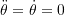, and let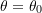. Therefore: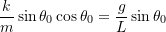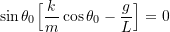Therefore. the static configurations are given by:Assuming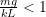, there are 3 positions.

To find the motion, linearize (1) about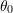. Set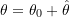, where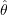is a small angle.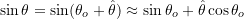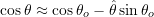Put the above into the original equation of motion to get: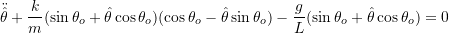Note that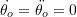.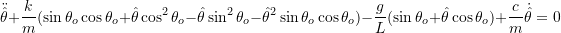Using the fact that the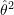term is small compared to 1, it becomes: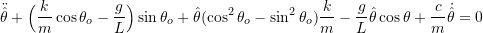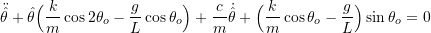The above equation looks like the standard form except for a constant term that is zero for all conditions.

Now consider all three positions:

1.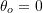.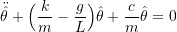Therefore, this is stable if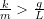as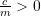, but unstable if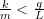.

2.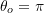.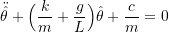Therefore, this is always stable.

3.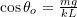.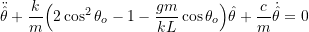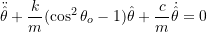Therefore, this is always unstable, regardless of damping.

###### Example

1.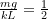,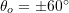,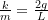. Therefore: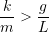2.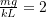,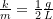. Therefore: